In this post I explore different tools and methods that can be used to try and interpret "black box" models.

Since the NFL draft is today, I thought a fun dataset to use for this topic would be the NFL combine data. Using that data we'll first build a model to predict the early career performance for NFL defensive ends (DE). After that, we will use different interpretability methods to better understand the model.

# Creating a model¶

Let's get started by importing what we need to set up the data and our modeling pipeline.

In :
%matplotlib inline

import matplotlib.pyplot as plt
import seaborn as sns
import numpy as np
import pandas as pd

from sklearn.preprocessing import Imputer
from sklearn.model_selection import  cross_val_score
from sklearn.pipeline import Pipeline
from sklearn.metrics import make_scorer
from sklearn.ensemble import RandomForestRegressor

from skll.metrics import spearman

from skopt import BayesSearchCV
from skopt.space import Real, Categorical, Integer

import warnings

In :
# setting up the styling for the plots in this notebook
sns.set(style="white", palette="colorblind", font_scale=1.2,
rc={"figure.figsize":(12,9)})
RANDOM_STATE = 420
N_JOBS=8


Here we load in the data that contains each player's combine information and their 3 year Approximate Value, which we will use as measure of early career performance (and going forward will be abbreviated as AV). When building our model we won't be predicting a defensive end's AV, instead we'll be predicting be where his AV ranks (as a percentile) among other defensive ends. Players with high a AV should be closer to 1, while players with low AV should be closer to 0.

NOTE: If you want read up on which combine measurables matter for each NFL position, take a look at Bill Lotter's series of blog posts on the topic.

In :
# read in our data
# onyl get players that have been in the league for 3 years
data_df2 = data_df.loc[data_df.Year <= 2015].copy()
# calculate the player AV percentiles by position
data_df2['AV_pctile'] = (data_df2.groupby('Pos')
.AV
.rank(pct=True,
method='min',
ascending=True))


Now that we've loaded the data and calculated the AV percentiles, let's get the DE data and create a training set and testing set. We will train and tune our model on the first 8 years (2000-2011) of combine data and then test it on the next 4 years (2012-2015).

In :
# Get the data for the position we want, in this case it's DE
pos_df = data_df2.loc[data_df2.Pos=='DE'].copy().reset_index(drop=True)

# Split the data into train and test sets
train_df = pos_df.loc[pos_df.Year <= 2011]
test_df = pos_df.loc[pos_df.Year.isin([2012, 2013, 2014, 2015])]


We'll be build a random forest model since that seems to be the most common "black box" algorithm people use at work. One thing to to note is that in our modeling Pipeline we will need to include an Imputer because some DEs are missing data as they did not participate in all the combine drills.

In :
# Combine measurables
features = ['Forty',
'Wt',
'Ht',
'Vertical',
'BenchReps',
'Cone',
'Shuttle']
# what we want to predict
target = 'AV_pctile'

X = train_df[features].values
y = train_df[target].values

# the modeling pipeline
pipe = Pipeline([("imputer", Imputer()),
("estimator", RandomForestRegressor(random_state=RANDOM_STATE))])


To tune our model we will use BayesSearchCV from scikit-optimize, which utilizes bayesian optimization to find the best hyperparameters. We'll use Spearman's rank correlation as our scoring metric since we are mainly concerned with the ranking of players when it comes to the draft.

In :
# We use spearman's rank correlation as the scoring metric since
# we are concerned with ranking the players
spearman_scorer = make_scorer(spearman)

# the hyperparamters to search over, including different imputation strategies
rf_param_space = {
'imputer__strategy': Categorical(['mean', 'median', 'most_frequent']),
'estimator__max_features': Integer(1, 8),
'estimator__n_estimators': Integer(50, 500),
'estimator__min_samples_split': Integer(2, 200),
}
# create our search object
search = BayesSearchCV(pipe,
rf_param_space,
cv=10,
n_jobs=N_JOBS,
verbose=0,
error_score=-9999,
scoring=spearman_scorer,
random_state=RANDOM_STATE,
return_train_score=True,
n_iter=75)
# fit the model
# I get some funky warnings, possibly due to the spearman scorer,
# I choose to suppress them
with warnings.catch_warnings():
warnings.filterwarnings('ignore')
search.fit(X, y)

In :
# best model parameters
search.best_params_

Out:
{'estimator__max_features': 6,
'estimator__min_samples_split': 63,
'estimator__n_estimators': 500,
'imputer__strategy': 'median'}
In :
# CV score
search.best_score_

Out:
0.38699012444314973
In :
# CV standard deviation
search.cv_results_['std_test_score'][search.best_index_]

Out:
0.13872941403084682

Now that we've tuned our model let's evaluate it on the test set.

In :
# test set data
X_test = test_df[features].values
y_test = test_df[target].values
# predictions
y_pred = search.predict(X_test)
# evaluation
model_test_score = spearman_scorer(search, X_test, y_test)
model_test_score

Out:
0.20929440372118885

So our model's predictions had a Spearman's rank correlation of about 0.209. Is that good or bad? I'm not sure. To get a better sense of how good or how bad of a score that is we can use the actual draft picks as a comparison point.

In :
# create percentiles for nfl draft picks
# Lower numerical picks (e.g. 1, 2, 3) are ranked closer to 1
# Higher numerical picks (e.g. 180, 200, etc) are ranked closer to 0
draft_pick_pctile = test_df.Pick.rank(pct=True,
method='min',
ascending=False,
na_option='top')
spearman(y_test, draft_pick_pctile)

Out:
0.6425823905799751

It looks like NFL teams are 3 times better at ranking DEs than our model.

Ok great, now that we have our model let's explore the different tools we can use to better understand it.

# Feature Importance¶

## Mean Decrease Impurity¶

When using a tree-ensemble like random forest you can find out which features the model found valuable by checking the feature importances. In scikit-learn the feature importances are a reflection of how well a feature reduces some criterion. In our regression example that criterion is mean squared error. This method for calculating feature importance is typically called mean decrease impurity or gini importance.

We can access our model's feature importances with the feature_importances_ attribute.

In :
# get the estimator and imputer from our pipeline, which will be used
# as we try and interpret our model
estimator = search.best_estimator_.named_steps['estimator']
imputer = search.best_estimator_.named_steps['imputer']

estimator.feature_importances_

Out:
array([0.34196584, 0.3615381 , 0.01465109, 0.03990757, 0.05417351,
0.05790136, 0.07712715, 0.05273537])

Alternatively, we could use eli5's explain_weights_df function, which returns the importances and the feature names we pass it as a pandas DataFrame.

In :
import eli5

# create our dataframe of feature importances
feat_imp_df = eli5.explain_weights_df(estimator, feature_names=features)
feat_imp_df

Out:
feature weight std
0 Wt 0.361538 0.111257
1 Forty 0.341966 0.129695
2 Cone 0.077127 0.106589
4 BenchReps 0.054174 0.081691
5 Shuttle 0.052735 0.079622
6 Vertical 0.039908 0.077301
7 Ht 0.014651 0.037999

So it looks like a player's weight and their forty time are important to model. A thing to note is that explain_weights_df also returns the standard deviations, but they may not be trustworthy as those values assume a normal distribution. Instead of relying on those standard deviations we can access each tree in our ensemble and plot the full distribution of feature importances.

In :
# get the feature importances from each tree and then visualize the
# distributions as boxplots
all_feat_imp_df = pd.DataFrame(data=[tree.feature_importances_ for tree in
estimator],
columns=features)

(sns.boxplot(data=all_feat_imp_df)
.set(title='Feature Importance Distributions',
ylabel='Importance'));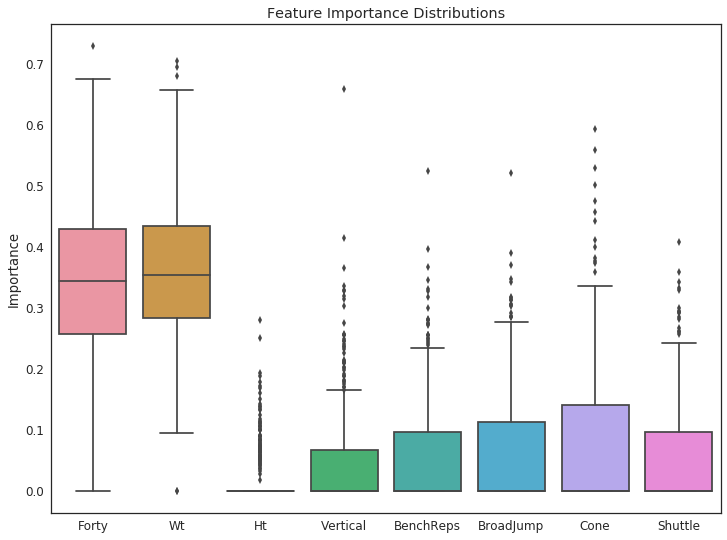## Permutation Importance¶

Permutation importances or mean decrease accuracy (MDA) is an alternative to mean decrease impurity that can be applied to any model. The basic idea of permutation importance is to permute the values of each feature and measure how much that permutation negatively impacts the scoring metric (which in our case is the Spearman's rank correlation). This gives us a sense of how our model would perform without that specific feature. All we need to do calculate permutation importance is use PermutationImportance from eli5.

In :
from eli5.sklearn import PermutationImportance

# we need to impute the data first before calculating permutation importance
train_X_imp = imputer.transform(X)
# set up the met-estimator to calculate permutation importance on our training
# data
perm_train = PermutationImportance(estimator, scoring=spearman_scorer,
n_iter=50, random_state=RANDOM_STATE)
# fit and see the permuation importances
perm_train.fit(train_X_imp, y)
eli5.explain_weights_df(perm_train, feature_names=features)

Out:
feature weight std
0 Wt 0.317768 0.038552
1 Forty 0.261409 0.036946
2 Cone 0.033025 0.006485
3 Shuttle 0.023978 0.005698
5 BenchReps 0.016859 0.004783
6 Vertical 0.012590 0.003537
7 Ht 0.005083 0.001254
In :
# plot the distributions
perm_train_feat_imp_df = pd.DataFrame(data=perm_train.results_,
columns=features)
(sns.boxplot(data=perm_train_feat_imp_df)
.set(title='Permutation Importance Distributions (training data)',
ylabel='Importance'));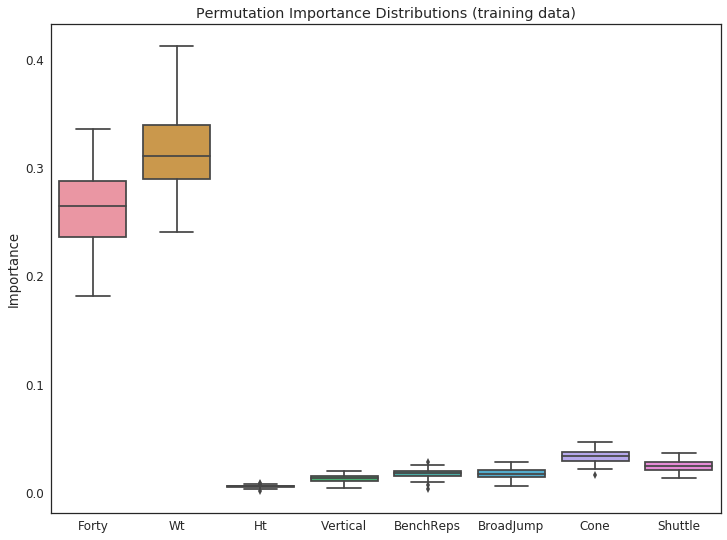Based on the permutation importances it again looks like Forty and Wt are the two most important features to the model.

# Feature Contributions¶

While feature importances can provide us insight into which variables a model finds valuable, they don't tell us how those features impact our model's predictions. One way to actually do that is by using the decision paths in our trees to see how much each feature changes the predictions when going from the parent to the child node. In the end we can break down these feature contributions in a linear manner such that our predictions can be interpreted like so:

$$prediction = bias + feature_{1}contribution + feature_{2}contribution +... feature_{n}contribution$$

Ando Saabas has written a few blog posts that delve into this topic. For now I will try to explain how feature contributions are calculated by looking at an example that uses a shallow tree in our forest.

In :
from IPython.display import Image
from sklearn.tree import export_graphviz
import graphviz
import pydotplus
from io import StringIO

# source for plotting decision tree
# https://medium.com/@rnbrown/creating-and-visualizing-decision-trees-with-python-f8e8fa394176
# Get all trees of depth 2 in the random forest
depths2 = [tree for tree in estimator.estimators_ if tree.tree_.max_depth==2]
# grab the first one
tree = depths2
# plot the tree
dot_data = StringIO()
export_graphviz(tree, out_file=dot_data, feature_names=features,
filled=True, rounded=True, special_characters=True)
graph = pydotplus.graph_from_dot_data(dot_data.getvalue())
Image(graph.create_png())

Out: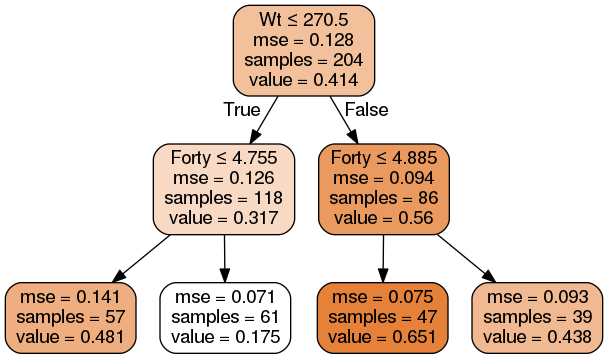To keep things simple let's find the feature contributions for a player that follows the leftmost path in the tree above, say a player who weighs 260 lbs and runs 4.6 seconds in the forty yard dash.

The player first starts at the root (top) node, where the mean AV percentile of all the samples is 0.414 (this is value of our bias term). At the first split, the tree considers whether the player is 270.5 lbs or less, so any change in the prediction caused by this split is attributed to the player's weight. Our player falls into the left child node because he weighs 260 lbs. Since the average percentile at this node is 0.317 versus 0.414 in the parent node, we can say that the player's weight caused a decrease of 0.097 percentage points in his predicted AV percentile. Now for the split at this new node, the tree considers whether the player runs a 4.755 forty or less. The player in our example runs a 4.6 forty, so after this split, he ends up in the leftmost leaf node of the tree. At this leaf node the player's final predicted percentile is 0.481, since that is an increase of 0.164 percentage points from the previous node, we can say that the player's forty time contributed 0.164 percentage points to his predicted AV percentile. In the end, the feature contributions for this player's predicted AV percentile are as follows:

$$\underset{\text{AV %ile}}{0.481} = \underset{\text{bias}}{0.414}-\underset{\text{Wt}}{0.097}+\underset{\text{Forty}}{0.164}$$

Which are the contribution values we get when we use eli5's explain_prediction_df function.

In :
# simple exmaple of a player with a 4.6 Forty and a Wt of 260 lbs
example = np.array([4.6, 260, 0, 0, 0, 0, 0, 0])
eli5.explain_prediction_df(tree, example, feature_names=features)

Out:
target feature weight value
0 y 0.413820 1.0
1 y Forty 0.163935 4.6
2 y Wt -0.097034 260.0

And 0.481 should be what the tree predicts.

In :
tree.predict(example.reshape(1,-1))

Out:
array([0.48072043])

Which it does. To calculate the bias term and feature contributions for the entire forest of trees, all you do is average the bias terms and feature contributions of all the trees.

NOTE: I'm aware of two libraries that allow you to easily calculate these kinds of feature contributions, eli5 and Ando Sabaas' treeintepretter. Besides for the difference in their APIs there are differences in which algorithms they can be used with. treeinterpretter currently works for the following scikit-learn estimators:

• DecisionTreeRegressor
• DecisionTreeClassifier
• ExtraTreeRegressor
• ExtraTreeClassifier
• RandomForestRegressor
• RandomForestClassifier
• ExtraTreesRegressor
• ExtraTreesClassifier

eli5 works for those estimator plus both of scikit-learn's gradient boosting estimators as well as XGBoost and LightGBM estimators.

Now let's actually get the feature contributions for each sample in our training and testing sets. One thing to note is that the explain_prediction_df only calculates the contributions one observation at a time, which can be time consuming. To speed things up I wrote a helper function that let's us use multiple processes (i.e. multiple cpu cores) to get the feature contributions for all our predictions.

In :
from concurrent.futures import ProcessPoolExecutor

def multiproc_iter_func(max_workers, an_iter, func, item_kwarg, **kwargs):
"""
A helper functions that applies a function to each item in an iterable using
multiple processes. 'item_kwarg' is the keyword argument for the item in the
iterable that we pass to the function.
"""
with ProcessPoolExecutor(max_workers=max_workers) as executor:
future_results = [executor.submit(func, **{item_kwarg: item}, **kwargs)
for item in an_iter]

results = [future.result() for future in future_results]

return results

In :
# Contibrutions for training set predictions
# construct a list of all contributions for the entire train set
train_expl_list = multiproc_iter_func(N_JOBS, train_X_imp,
eli5.explain_prediction_df, 'doc',
estimator=estimator,
feature_names=features)
# concatenate them into 1 large dataframe, with the proper player name as an
# index
train_expl_df = pd.concat(train_expl_list, keys=train_df.Player,
names=['Player'])
# take a look at a couple of players

Out:
target feature weight value
Player
Michael Boireau 0 y 0.421925 1.000
1 y Wt 0.050163 274.000
2 y BenchReps 0.005912 26.000
3 y Ht -0.000552 76.000
4 y Shuttle -0.003833 4.490
5 y Vertical -0.004394 29.000
7 y Cone -0.010553 7.680
8 y Forty -0.103266 5.090
Courtney Brown 0 y 0.421925 1.000
1 y Forty 0.020799 4.780
2 y BenchReps 0.010175 24.000
3 y Shuttle 0.005032 4.410
4 y Cone -0.000513 7.365
6 y Ht -0.003440 77.000
7 y Vertical -0.004461 33.000
8 y Wt -0.046967 269.000
In :
# Contributions for test set predictions
# we need to impute the missing values in the test set
test_X_imp = imputer.transform(X_test)
# now repeat what we did with the training data on the test data
test_expl_list = multiproc_iter_func(N_JOBS, test_X_imp,
eli5.explain_prediction_df, 'doc',
estimator=estimator,
feature_names=features)

test_expl_df = pd.concat(test_expl_list, keys=test_df.Player,
names=['Player'])

Out:
target feature weight value
Player
Frank Alexander 0 y 0.421925 1.000
1 y Forty 0.025510 4.800
2 y BenchReps 0.011177 24.000
3 y Shuttle 0.001877 4.410
4 y Wt 0.001765 270.000
5 y Cone 0.000624 7.365
7 y Ht -0.002257 76.000
8 y Vertical -0.004324 33.000
Jake Bequette 0 y 0.421925 1.000
1 y Wt 0.063637 274.000
2 y Forty 0.041246 4.750
3 y Cone 0.035639 6.900
4 y Shuttle 0.015801 4.070
5 y BenchReps 0.010608 24.000
6 y Vertical 0.001309 34.000
7 y Ht -0.001039 77.000
In :
# Double check that the sums of contributions equal the actual predictions
y_pred_sums = test_expl_df.groupby('Player').weight.sum()
np.allclose(y_pred, y_pred_sums)

Out:
True

## Plotting feature contributions¶

Now that we have all these feature contributions let's make some plots to better understand them.

### Boxplots¶

First let's make a boxplot to see the contribution distributions of each feature.

In :
# I"m creating one big dataframe that includes both train and test
# to plot them on same plot using seaborn's boxplot
train_expl_df.rename(columns={'weight': 'contribution'}, inplace=True)
test_expl_df.rename(columns={'weight': 'contribution'}, inplace=True)
train_expl_df['data'] = 'train'
test_expl_df['data'] = 'test'
train_test_expl_df = pd.concat([train_expl_df, test_expl_df])
sns.boxplot(x='feature', y='contribution', hue='data', order=features,
data=train_test_expl_df.loc[train_test_expl_df.feature!=''],
palette={'train': 'salmon',
'test':'deepskyblue'})
plt.legend(loc=9)
plt.title('Distributions of Feature Contributions');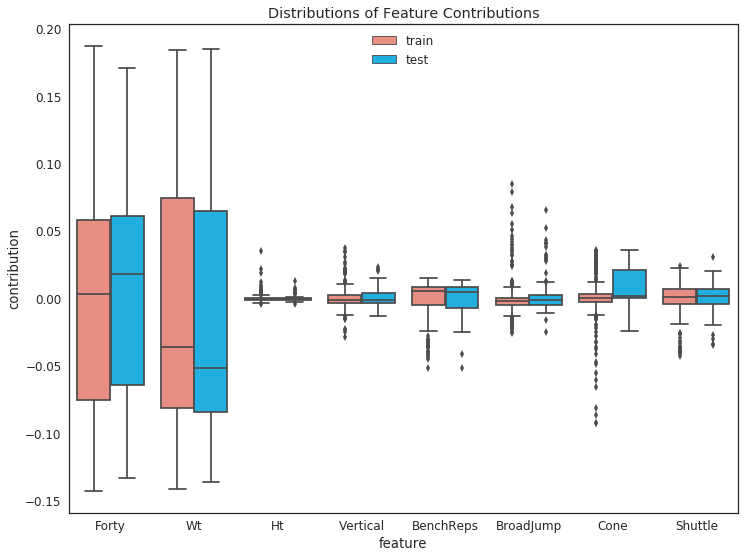### Swarmplots¶

We can also use swarmplots which allow for a more granular view of the distribution since they plot each individual observation. If we color each point by the associated feature's (scaled) value, we can view how the change in a feature's value changes along with its contribution.

seaborn doesn't support a colorbar by default so it's something we have to add on our own. I hacked together function (based on this stackoverflow answer) that will let us add a vertical colorbar to the right of our swarmplots.

In :
from matplotlib.colorbar import ColorbarBase
from mpl_toolkits.axes_grid1 import make_axes_locatable

In :
# a hacky function that plots a swarmplot along with a colorbar
# based off the code found here:
# https://stackoverflow.com/questions/40814612/map-data-points-to-colormap-with-seaborn-swarmplot
def swarmplot_with_cbar(cmap, cbar_label, *args, **kwargs):
fig = plt.gcf()
ax = sns.swarmplot(*args, **kwargs)
# remove the legend, because we want to set a colorbar instead
ax.legend().remove()
## create colorbar ##
divider = make_axes_locatable(ax)
cb = ColorbarBase(ax_cb, cmap=cmap, orientation='vertical')

return fig

In :
# min-max scaling of the feature values allows us to use a colorbar
# to indicate high or low feature values
train_scaled_feat_vals = (train_expl_df.groupby('feature')
.value
.transform(lambda x: x/x.max()))

train_expl_df['scaled_feat_vals'] = train_scaled_feat_vals

cmap = plt.get_cmap('viridis')
cbar_label = 'Feature Value %ile'

plt.title('Distribution of Feature Contributions (training data)')
swarmplot_with_cbar(cmap, cbar_label,  x='feature', y='contribution',
hue='scaled_feat_vals', palette='viridis', order=features,
data=train_expl_df.loc[train_expl_df.feature!='']);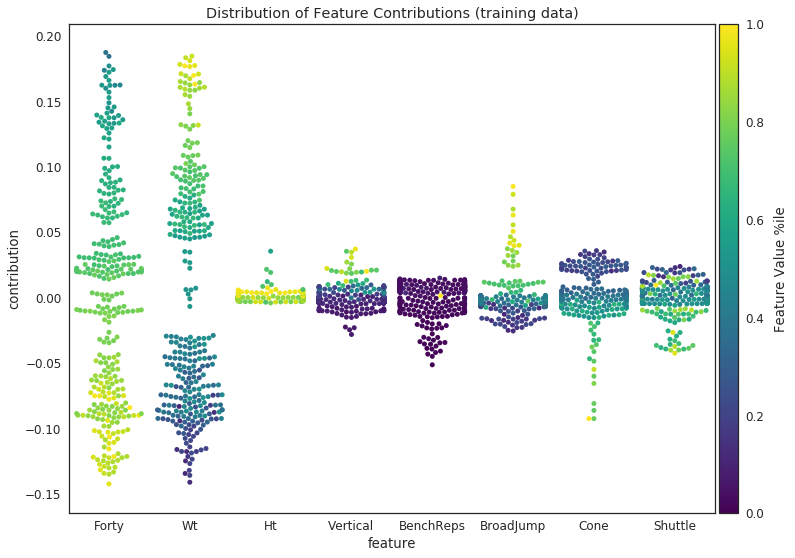In :
test_scaled_feat_vals = (test_expl_df.groupby('feature')
.value
.transform(lambda x: x/x.max()))

test_expl_df['scaled_feat_vals'] = test_scaled_feat_vals

plt.title('Distribution of Feature Contributions (test data)')
swarmplot_with_cbar(cmap, cbar_label,  x='feature', y='contribution',
hue='scaled_feat_vals', palette='viridis', order=features,
data=test_expl_df.loc[test_expl_df.feature!='']);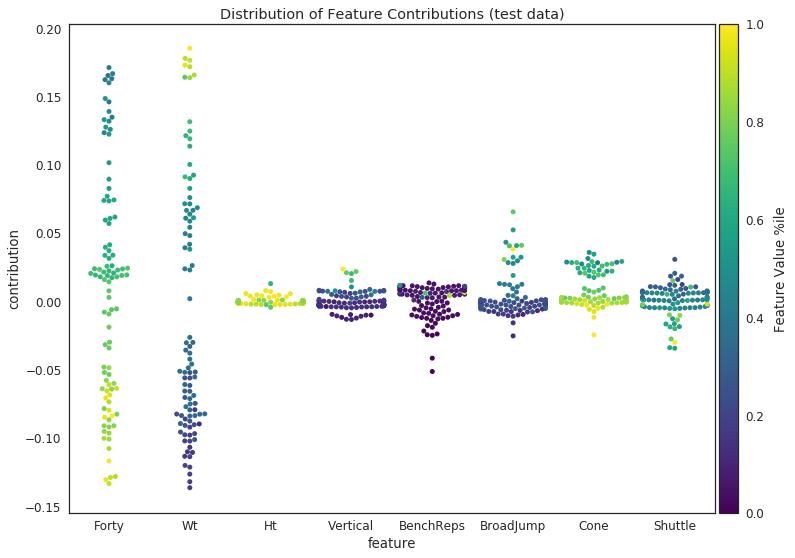Based on both plots, we can see that faster forty times and higher weights result in more positive contributions. The other features tend to have most of their contributions hover around 0. It's also interest to note the gaps in middle of the Wt distributions on both plots.

### Plotting Feature Contributions against Feature Values¶

Let's plot the feature contributions against the feature values to get a better sense of how they relate to one another. We can use seaborn's lmplot to easily create a grid of these kinds of plots for both our training and testing data.

In :
fg = sns.lmplot(x='value', y='contribution', col='feature',
data=train_expl_df.loc[train_expl_df.feature!=''],
col_order=features, sharex=False, col_wrap=3, fit_reg=False,
size=4, scatter_kws={'color':'salmon', 'alpha': 0.5, 's':30})
fg.fig.suptitle('Feature Contributions vs Feature Values (training data)')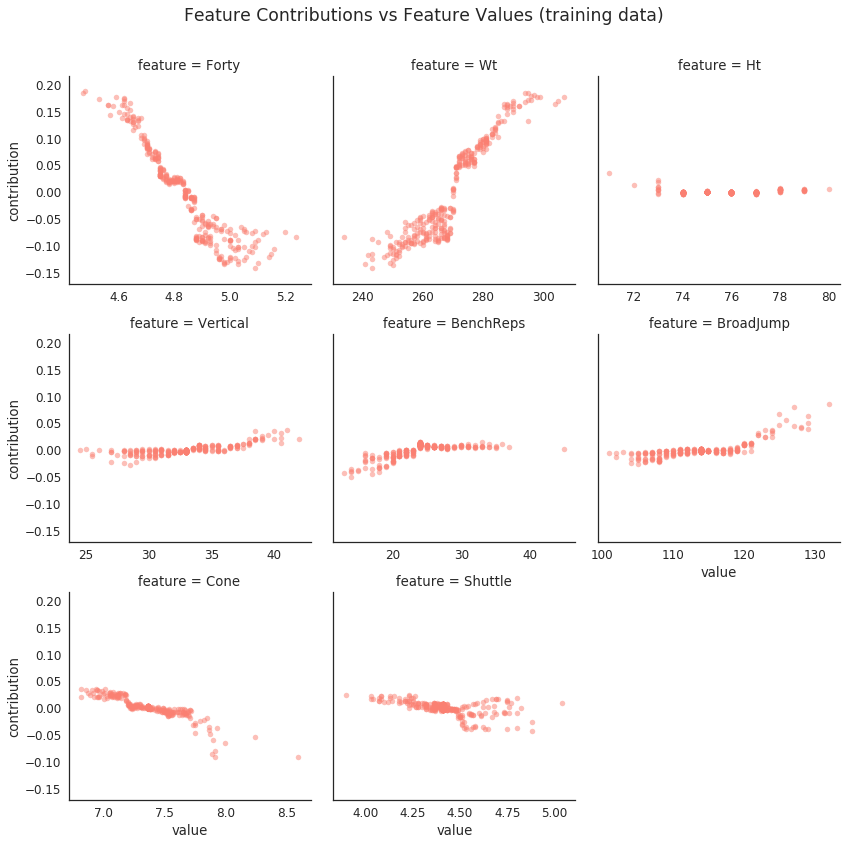In :
fg = sns.lmplot(x='value', y='contribution', col='feature',
data=test_expl_df.loc[test_expl_df.feature!=''],
col_order=features, sharex=False, col_wrap=3, fit_reg=False,
size=4, scatter_kws={'color':'salmon', 'alpha': 0.5, 's':30})
fg.fig.suptitle('Feature Contributions vs Feature Values (testing data)')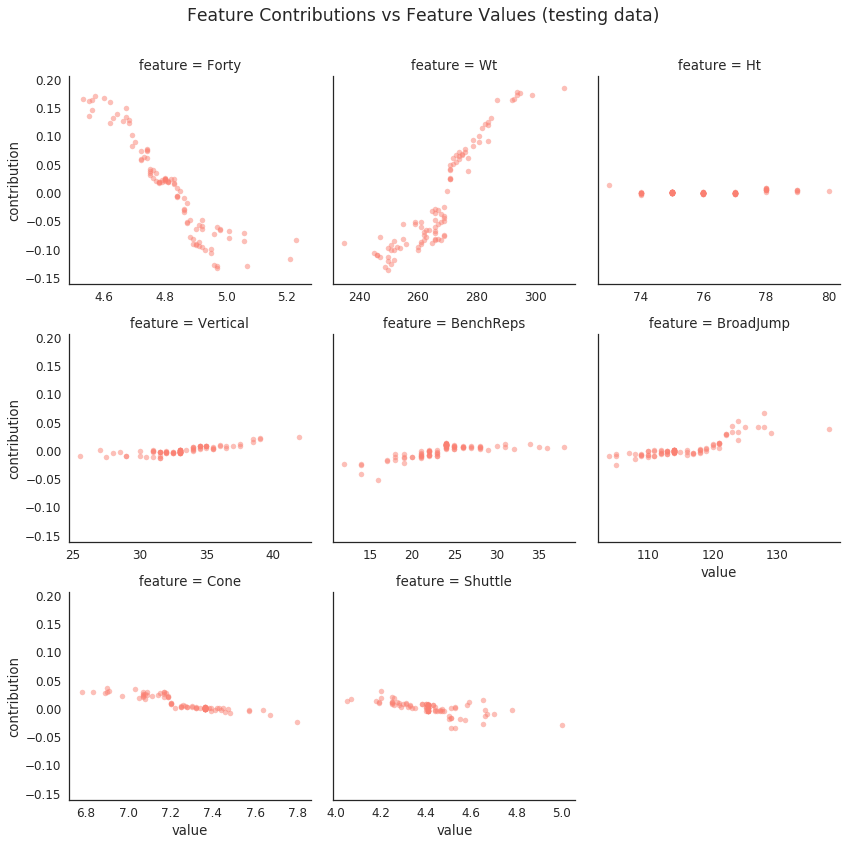In both plots for Wt it's interesting to see the rapid increase in contribution at around 270 lbs. The model essentially believes that weighing more than 270 is automatically a positive factor for a player, while weighing less than that is a negative one.

One thing to note about these plots is that when we see different contributions (e.g. -0.05, -0.10, -0.15) for the same feature value (e.g. a forty time of 5 seconds) there is probably another feature (or set of features) that is causing these differences. To view such feature interactions we can set the color of the dots to reflect the value of another feature. Let's take a look at how a player's weight interacts with the contribution of their forty time (at least in the training set).

In :
# before we actually plot anything we need to do a bit of data manipulation
# let's pivot the data and create a new dataframe where the columns are
# the feature contributions and each row is a player, with the player
# name as the index value
# here are different ways to pivot column values to columns
# https://stackoverflow.com/questions/26255671/pandas-column-values-to-columns
# based on running %%timeit, the groupby method was fastest
train_contrib_df = (train_expl_df.groupby(['Player','feature'])
.contribution
.aggregate('first')
.unstack())
# add in the feature values
train_feat_contrib_df = train_contrib_df.merge(train_df[['Player'] + features],
how='left', left_index=True,
right_on='Player',
suffixes=('_contrib', '_value'))
# now we can plot
plt.scatter(x='Forty_value', y='Forty_contrib', c='Wt_value', cmap=cmap,
data=train_feat_contrib_df)
plt.xlabel('Forty')
plt.ylabel('contribution')
plt.colorbar(label='Wt');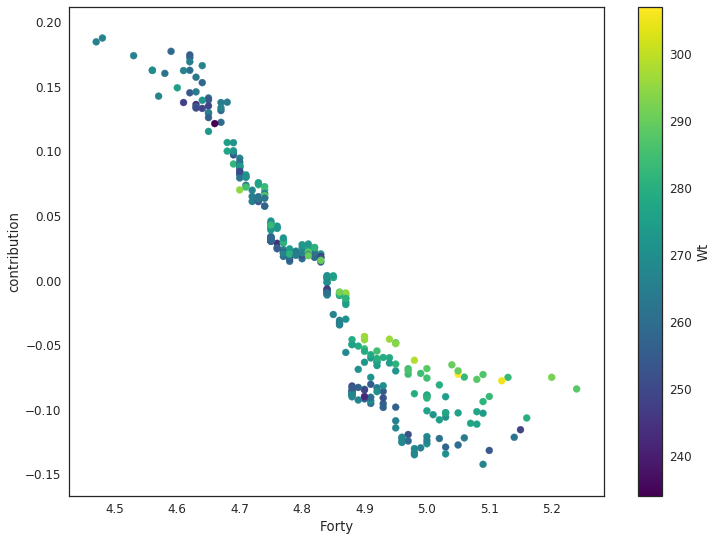We can see that there is some interaction between weight and forty time. Given a specific forty time, players with higher weights tend to have a more positive (or less negative) contribution.

### Heatmaps¶

With a little data wrangling and seaborn's heatmap function we can take a look at the full set of contributions for each player in our test set.

In :
def double_heatmap(data1, data2, cbar_label1, cbar_label2,
title='', subplot_top=0.86, cmap1='viridis', cmap2='magma',
center1=0.5, center2=0, grid_height_ratios=[1,4],
figsize=(14,10)):
# do the actual plotting
# here we plot 2 seperate heatmaps one for the predictions and actual percentiles
# the other for the contributions
# the reason I chose to do this is because of the difference in magnitudes
# between the percentiles and the contributions
fig, (ax,ax2) = plt.subplots(nrows=2, figsize=figsize,
gridspec_kw={'height_ratios':grid_height_ratios})

fig.suptitle(title)

# heatmap for actual and predicted percentiles
sns.heatmap(data1, cmap="viridis", ax=ax, xticklabels=False, center=center1,
cbar_kws={'location':'top',
'use_gridspec':False,
'label': cbar_label1})
ax.set_xlabel('')

# heatmap of the feature contributions
sns.heatmap(data2, ax=ax2, xticklabels=False, center=center2, cmap=cmap2,
cbar_kws={'location':'bottom',
'use_gridspec':False,
'shrink':0.41,
'label': cbar_label2})
ax2.set_ylabel('');
return fig

In :
# get the prediction and actual target values to plot
y_test_and_pred_df = pd.DataFrame(np.column_stack((y_test, y_pred)),
index=test_df.Player,
columns=['true_AV_pctile', 'pred_AV_pctile'])

# let's pivot the data such that the feature contributions are the columns
test_heatmap_df = (test_expl_df.groupby(['Player','feature'])
.contribution
.aggregate('first')
.unstack())

# there may be some NaNs if a feature did not contribute to a prediction,
# so fill them in with 0s
test_heatmap_df = test_heatmap_df.fillna(0)

# merge our predictions with the the contributions
test_heatmap_df = test_heatmap_df.merge(y_test_and_pred_df, how='left',
right_index=True, left_index=True)
# sort by predictions
test_heatmap_df.sort_values('pred_AV_pctile', ascending=True, inplace=True)

In :
title = 'Feature contributions to predicted AV %ile \nfor each player in the testing data'
fig = double_heatmap(test_heatmap_df[['true_AV_pctile', 'pred_AV_pctile']].T,
test_heatmap_df[features].T, '%ile', 'contribution',
title=title)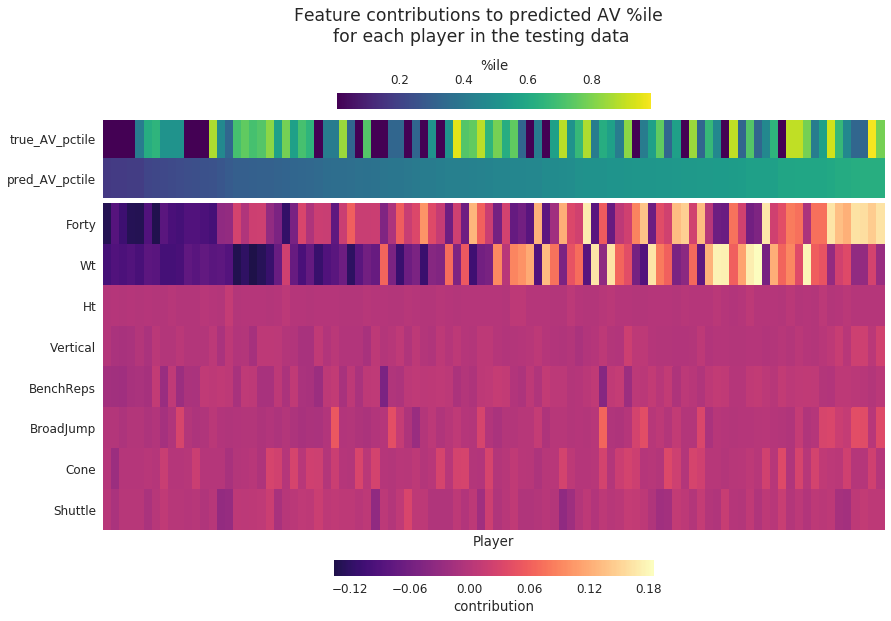The plot above consist of two heatmaps. Each column in both heatmaps represents a player. The rows in the top heatmap represent the true and predicted AV percentiles while the rows in the bottom heatmap represent the the feature contributions for each player prediction.

I like the above visualization because it makes it easy to view a large set of predictions and contributions all at once. I definitely prefer it over the alternative of viewing each set of contributions through a printout out of the DataFrame.

# Joint Feature Contributions¶

A benefit of using a tree-ensemble like our random forest model, is that it captures interactions among our features without us explicitly defining them. However in our attempt to interpret our model we have only looked at the importances and contributions of individual features. Since we've yet to measure the impact of the feature interactions that the model has found, we have an incomplete picture of what our model is actually doing. To gain some insight into these feature interactions we can use the treeinterpeter package. It uses the same method as before to calculate contributions, but instead of crediting individual features along the decision paths, treeinterpeter allows us to credit the feature interactions.

NOTE: If you use XGBoost you can use the xgbfir package to inspect feature interactions.

Let's use treeinterpreter on our simple decision tree from before, in order to get an idea of of how joint feature contributions (i.e. the contributions of the feature interactions) are calculated.

In :
# a reminder of what the tree looks like
Image(graph.create_png())

Out:To get the joint feature contributions, we pass in our estimator and the data to the predict function and set joint_contribution to True. That should return the prediction, the bias term and the joint feature contributions for our player.

In :
import treeinterpreter.treeinterpreter as ti
# get the contributions for our simple player example
# who has a 4.6 forty and weighs 260 lbs
# joint_contribution=True gets the joint feature contributions
# when set to False it just returns the individual feature contributions
example_pred, example_bias, example_contrib = ti.predict(tree,
example.reshape(1, -1),
joint_contribution=True)

In :
# same prediction as before
example_pred

Out:
array([0.48072043])
In :
# same bias value as before
example_bias

Out:
array([0.41381959])
In :
example_contrib

Out:
[{(0, 1): 0.163934535699354, (1,): -0.09703369274852375}]

The joint contributions are returned as list of dictionaries (in our example one dictionary for our one player), where the keys are numeric tuples representing the features (0 represents Forty and 1 represent Wt) and the values are the joint feature contributions. The joint feature contributions for our simple example are as follows:

$$\underset{\text{AV %ile}}{0.481} = \underset{\text{bias}}{0.414}-\underset{\text{Wt}}{0.097}+\underset{\text{Forty & Wt}}{0.164}$$

The contributions are the same values as before, the difference we see here is that instead of crediting the contribution of 0.164 percentage points to just the player's forty time, we also credit the previous feature, Wt, in the decision path. Remember, we are now crediting feature interactions, not just individual features. We only credit a single feature when it's either at the root node (like Wt is) or if it's the only feature used along a decision path.

Let's get the joint feature contributions for the test set predictions.

In :
joint_pred, joint_bias, joint_contrib = ti.predict(estimator,
test_X_imp,
joint_contribution=True)

In :
# double check predictions are correct
np.allclose(y_pred, joint_pred)

Out:
True
In :
# the bias is still the same
joint_bias[:3]

Out:
array([0.42192522, 0.42192522, 0.42192522])
In :
# 96 observations in test set
len(joint_contrib)

Out:
96
In :
# tuples representing the column indexes of our features
list(joint_contrib.keys())[:3]

Out:
[(0, 1), (0, 1, 2, 5), (0, 1, 5)]
In :
# an example of a joint feature contribution
joint_contrib[(0, 1)]

Out:
0.01733594064297664

To get the joint feature contributions into more useable format let's match the tuples of indexes to the proper feature names. Then we can construct a DataFrame of each player's joint feature contributions, ordered by the absolute value of the contributions.

In :
def create_ordered_joint_contrib_df(contrib):
"""
Creates a dataframe from the joint contribution info, where the
feature combinations are ordered (in descending fashion) by the absolute
value of the joint contribution.
"""
df = pd.DataFrame(contrib, columns=['feat_interaction', 'contribution'])
# get the reordered index
new_idx = (df.contribution.abs()
.sort_values(inplace=False, ascending=False)
.index)
df = df.reindex(new_idx).reset_index(drop=True)
return df

# add the names of the feats to the joint contributions
joint_contrib_w_feat_names = []
# for each observation in the join contributions
for obs in joint_contrib:
# create a list
obs_contrib = []
# for each tuple of column indexes
for k in obs.keys():
# get the associated feature names
feature_combo = [features[i] for i in k]
# get the contribution value
contrib = obs[k]
# store that information in the observation individual list
obs_contrib.append([feature_combo, contrib])
# append that individual to the large list containing each observations
# joint feature contributions
joint_contrib_w_feat_names.append(obs_contrib)

# create an ordered dataframe for each player
joint_contrib_dfs = [create_ordered_joint_contrib_df(contrib)
for contrib in joint_contrib_w_feat_names]
# now combine them all
joint_contrib_df = pd.concat(joint_contrib_dfs, keys=test_df.Player, names=['Player'])

# edit feat_interaction column so the values are strings and not lists
joint_contrib_df['feat_interaction'] = joint_contrib_df.feat_interaction.apply(' | '.join)

In :
joint_contrib_df.head()

Out:
feat_interaction contribution
Player
Frank Alexander 0 Forty | Wt 0.017336
1 Forty 0.010549
2 Wt -0.005477
3 Forty | Wt | BenchReps 0.003433
4 Forty | Wt | Shuttle 0.002922

Great, now we have the joint feature contributions for each player in our test set in a nice DataFrame. Let's take a look at how important each feature and feature interaction is to our predictions. To do that we will measure (as a percentage) how much of the total joint contributions an individual feature or feature interaction is responsible for. In other words we are going to measure the relative importance of each feature and feature interaction.

In :
# first get the sum of the absolute values for each joint feature contribution
abs_imp_joint_contrib = (joint_contrib_df.groupby('feat_interaction')
.contribution
.apply(lambda x: x.abs().sum())
.sort_values(ascending=False))

# then calculate the % of total contribution by dividing by the sum of all absolute vals
rel_imp_join_contrib = abs_imp_joint_contrib / abs_imp_joint_contrib.sum()